## Equilateral Triangle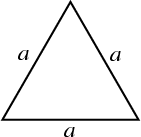An equilateral triangle is a Triangle with all three sides of equal length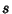. An equilateral triangle also has three equal 60° Angles.

An equilateral triangle can be constructed by Trisecting all three Angles of any Triangle (Morley's Theorem). Napoleon's Theorem states that if three equilateral triangles are drawn on the Legs of any Triangle (either all drawn inwards or outwards) and the centers of these triangles are connected, the result is another equilateral triangle.

Given the distances of a point from the three corners of an equilateral triangle,,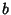, and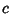, the length of a sideis given by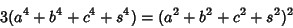(1)

(Gardner 1977, pp. 56-57 and 63). There are infinitely many solutions for which,, andare Integers. In these cases, one of,,, andis Divisible by 3, one by 5, one by 7, and one by 8 (Guy 1994, p. 183).

The Altitude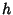of an equilateral triangle is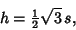(2)

whereis the side length, so the Area is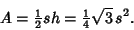(3)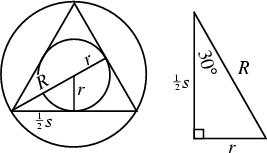The Inradius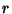, Circumradius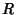, and Areacan be computed directly from the formulas for a general regular Polygon with side lengthand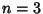sides,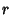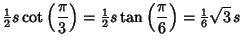(4)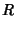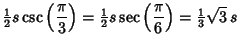(5)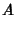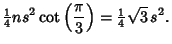(6)

The Areas of the Incircle and Circumcircle are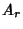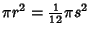(7)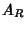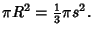(8)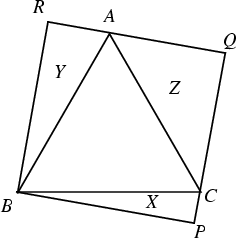Let any Rectangle be circumscribed about an Equilateral Triangle. Then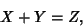(9)

where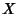,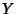, and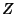are the Areas of the triangles in the figure (Honsberger 1985).

Begin with an arbitrary Triangle and find the Excentral Triangle. Then find the Excentral Triangle of that triangle, and so on. Then the resulting triangle approaches an equilateral triangle. The only Rational Triangle is the equilateral triangle (Conway and Guy 1996). A Polyhedron composed of only equilateral triangles is known as a Deltahedron.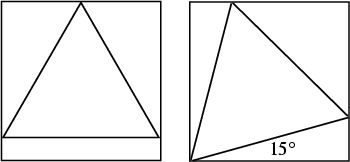The smallest equilateral triangle which can be inscribed in a Unit Square (left figure) has side length and area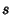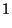(10)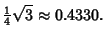(11)

The largest equilateral triangle which can be inscribed (right figure) is oriented at an angle of 15° and has side length and area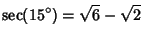(12)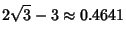(13)

See also Acute Triangle, Deltahedron, Equilic Quadrilateral, Fermat Point, Gyroelongated Square Dipyramid, Icosahedron, Isogonic Centers, Isosceles Triangle, Morley's Theorem, Octahedron, Pentagonal Dipyramid, Right Triangle, Scalene Triangle, Snub Disphenoid, Tetrahedron, Triangle, Triangular Dipyramid, Triaugmented Triangular Prism, Viviani's Theorem

References

Beyer, W. H. (Ed.) CRC Standard Mathematical Tables, 28th ed. Boca Raton, FL: CRC Press, p. 121, 1987.

Conway, J. H. and Guy, R. K. The Only Rational Triangle.'' In The Book of Numbers. New York: Springer-Verlag, pp. 201 and 228-239, 1996.

Dixon, R. Mathographics. New York: Dover, p. 33, 1991.

Gardner, M. Mathematical Carnival: A New Round-Up of Tantalizers and Puzzles from Scientific American. New York: Vintage Books, 1977.

Guy, R. K. Rational Distances from the Corners of a Square.'' §D19 in Unsolved Problems in Number Theory, 2nd ed. New York: Springer-Verlag, pp. 181-185, 1994.

Honsberger, R. Equilateral Triangles.'' Ch. 3 in Mathematical Gems I. Washington, DC: Math. Assoc. Amer., 1973.

Honsberger, R. Mathematical Gems III. Washington, DC: Math. Assoc. Amer., pp. 19-21, 1985.

Madachy, J. S. Madachy's Mathematical Recreations. New York: Dover, pp. 115 and 129-131, 1979.